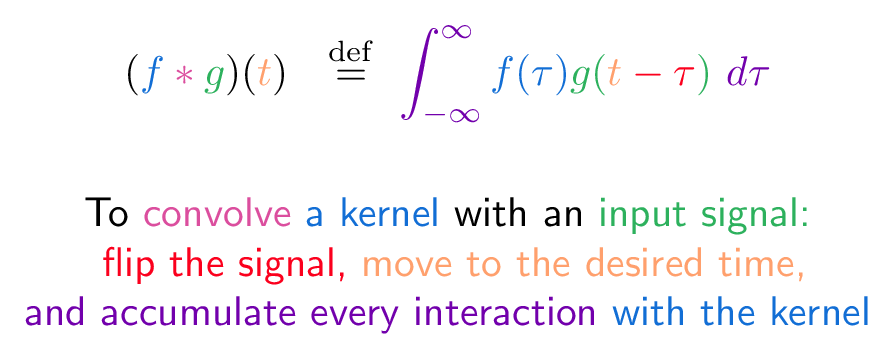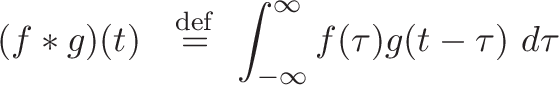# Convolution

## Colorized Definition\newcommand{\integral}{\color{c1}}
\newcommand{\signal}{\color{c2}}
\newcommand{\flip}{\color{c3}}
\newcommand{\kernel}{\color{c4}}
\newcommand{\slide}{\color{c5}}
\newcommand{\convolve}{\color{c6}}$\displaystyle{ (\kernel f \convolve * \signal g \plain )( \slide t \plain ) \ \ \stackrel{\mathrm{def}}{=}\ \integral \int_{-\infty}^\infty \kernel f(\tau) \signal g(\slide t \plain \flip - \tau \signal )\ \integral d\tau }$

\plain     To
\convolve  convolve
\kernel    a kernel
\plain     with an
\signal    input signal:
\\
\flip     flip the signal,
\slide    move to the desired time,
\
\integral and accumulate every interaction
\kernel with the kernel